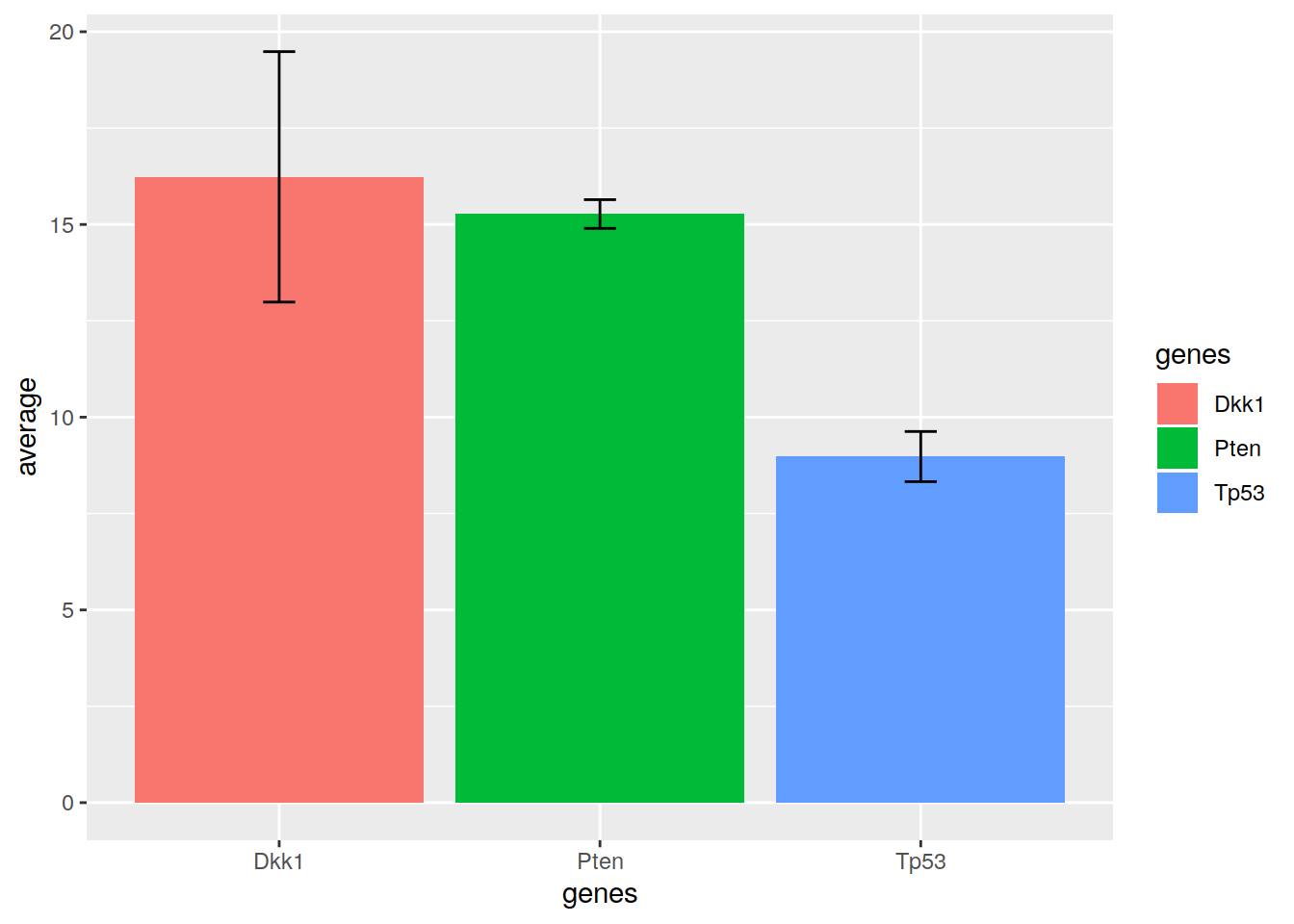## 19.5 Bar plots

``````# A simple bar plot
ggplot(data=df2, mapping=aes(x=grouping)) + geom_bar()``````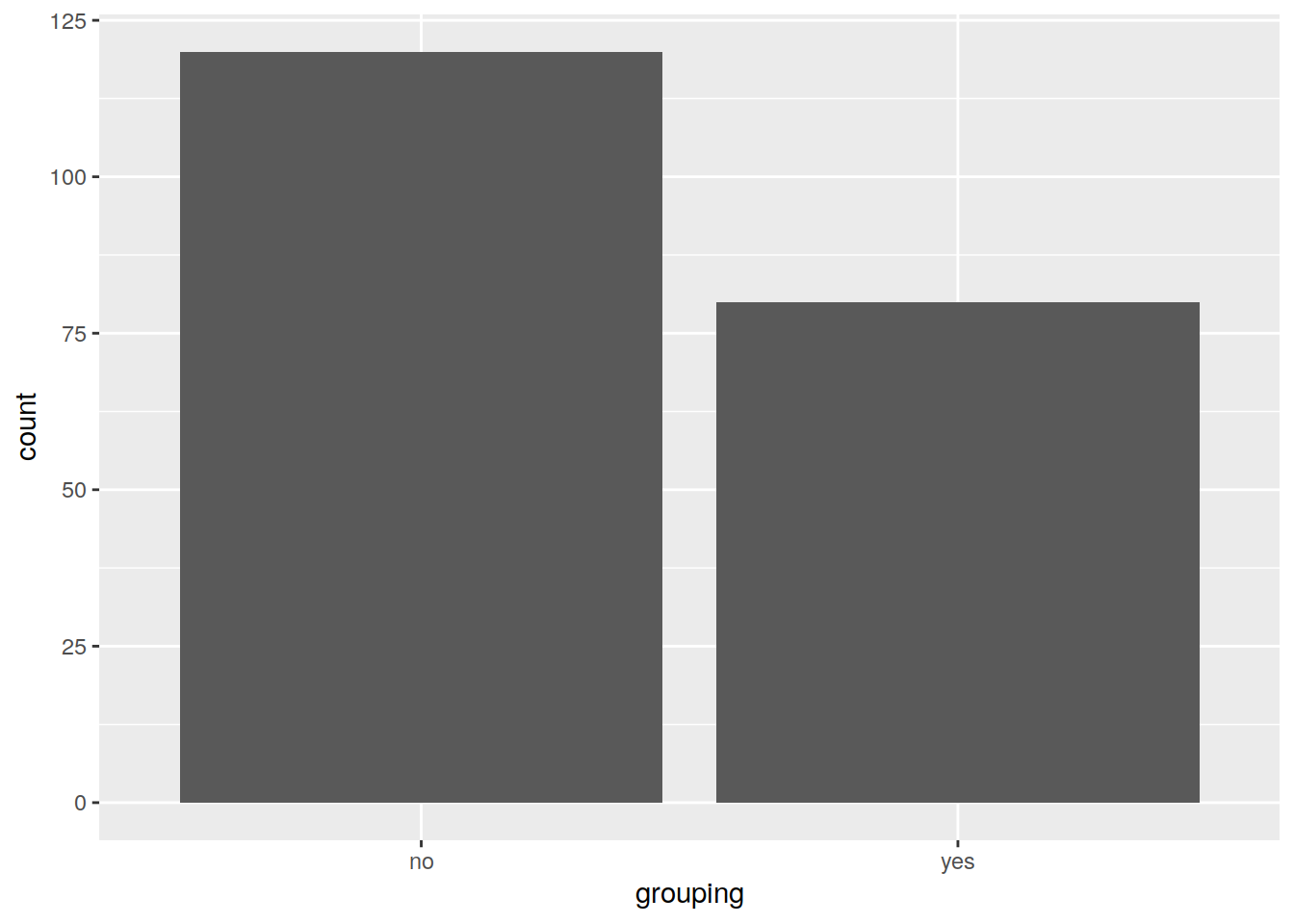• Customize:
• scale_x_discrete is used to handle x-axis title and labels
• coord_flip swaps the x and y axis
``````# Save the plot in the object "p"
pbar <- ggplot(data=df2, mapping=aes(x=grouping, fill=grouping)) +
geom_bar()

pbar``````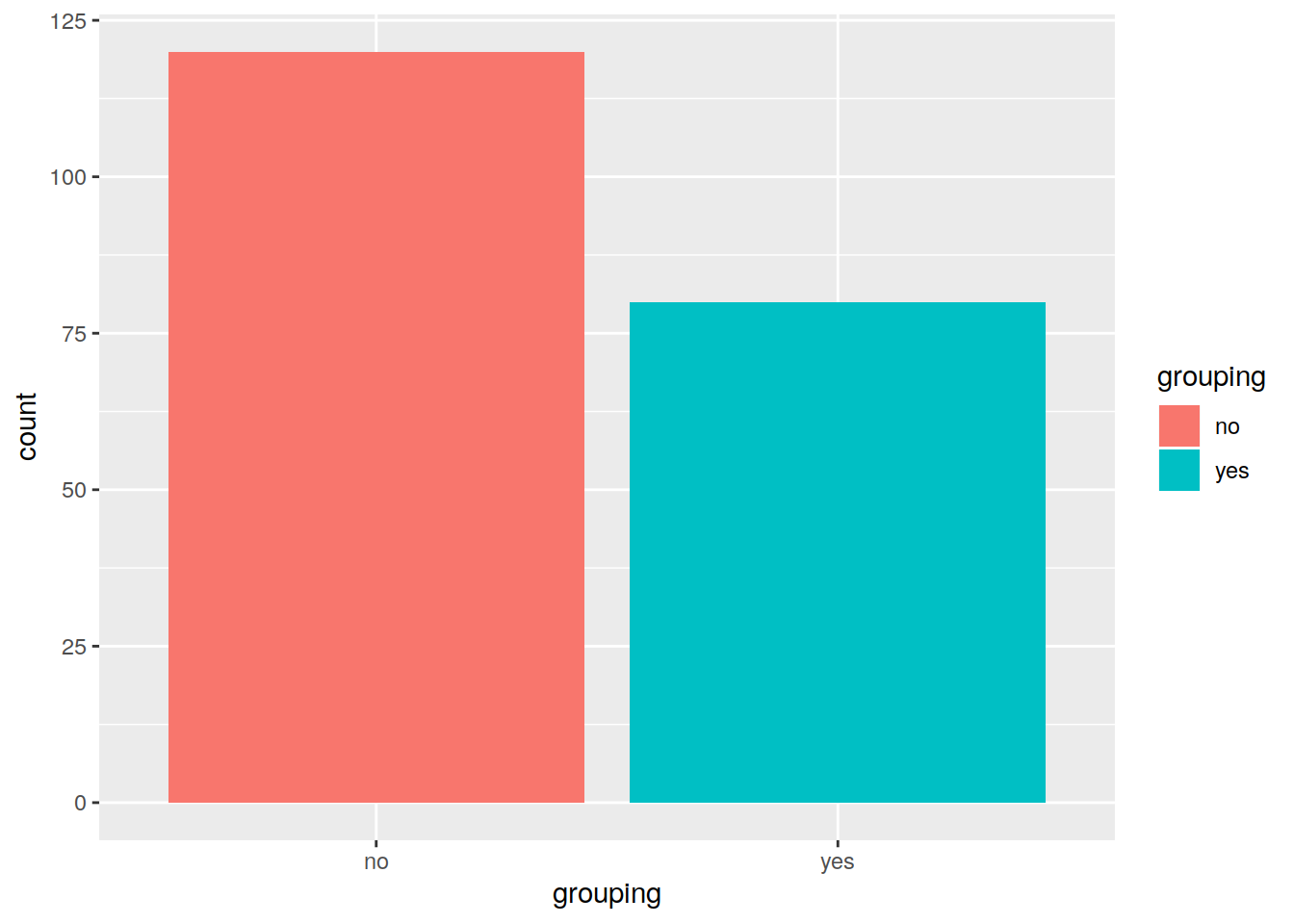``````# Change x axis label with scale_x_discrete and change order of the bars:
p2 <- pbar + scale_x_discrete(name="counts of yes / no", limits=c("yes", "no"))

p2``````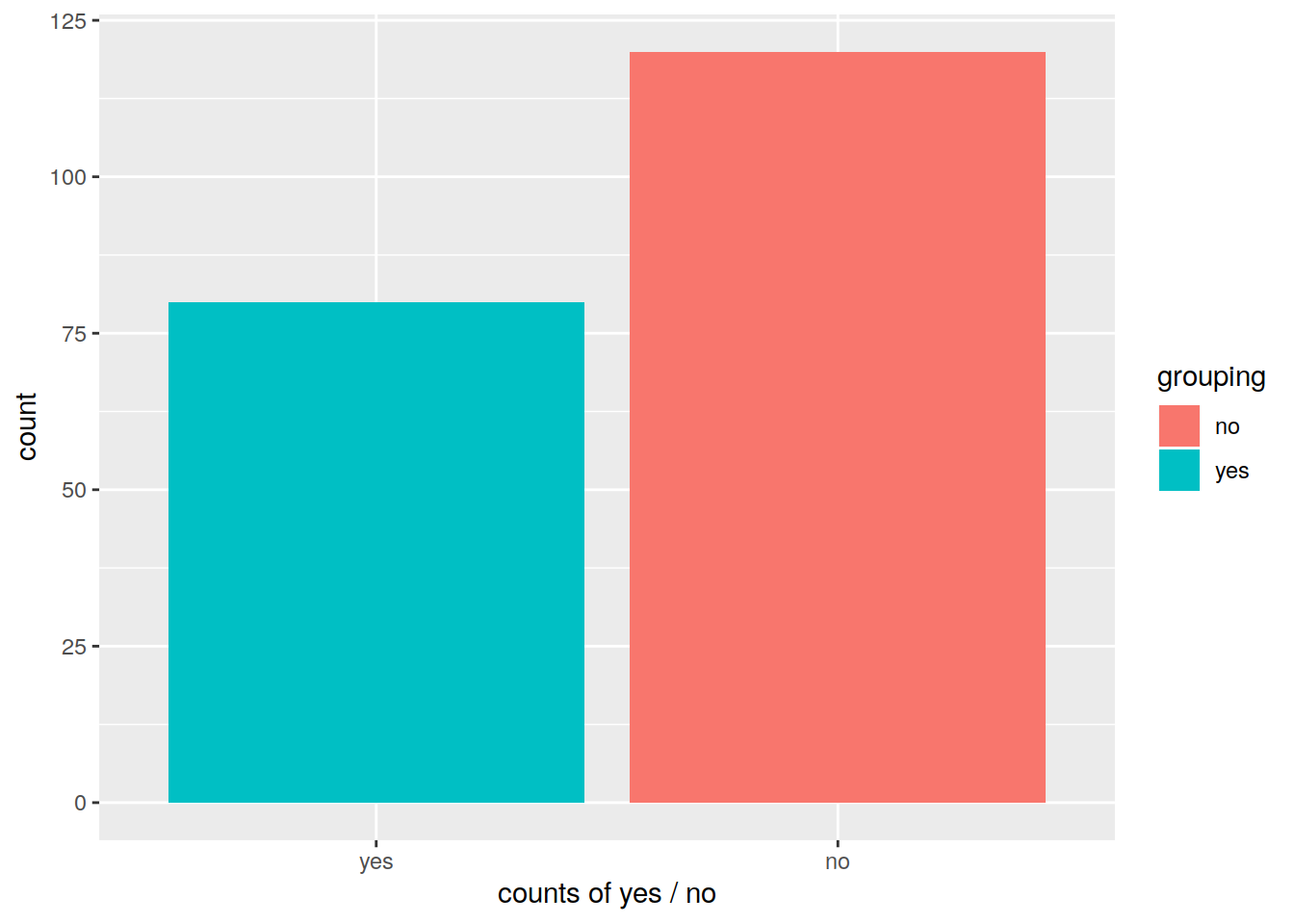``````# Swapping x and y axis with coord_flip():
p3 <- p2 + coord_flip()

p3``````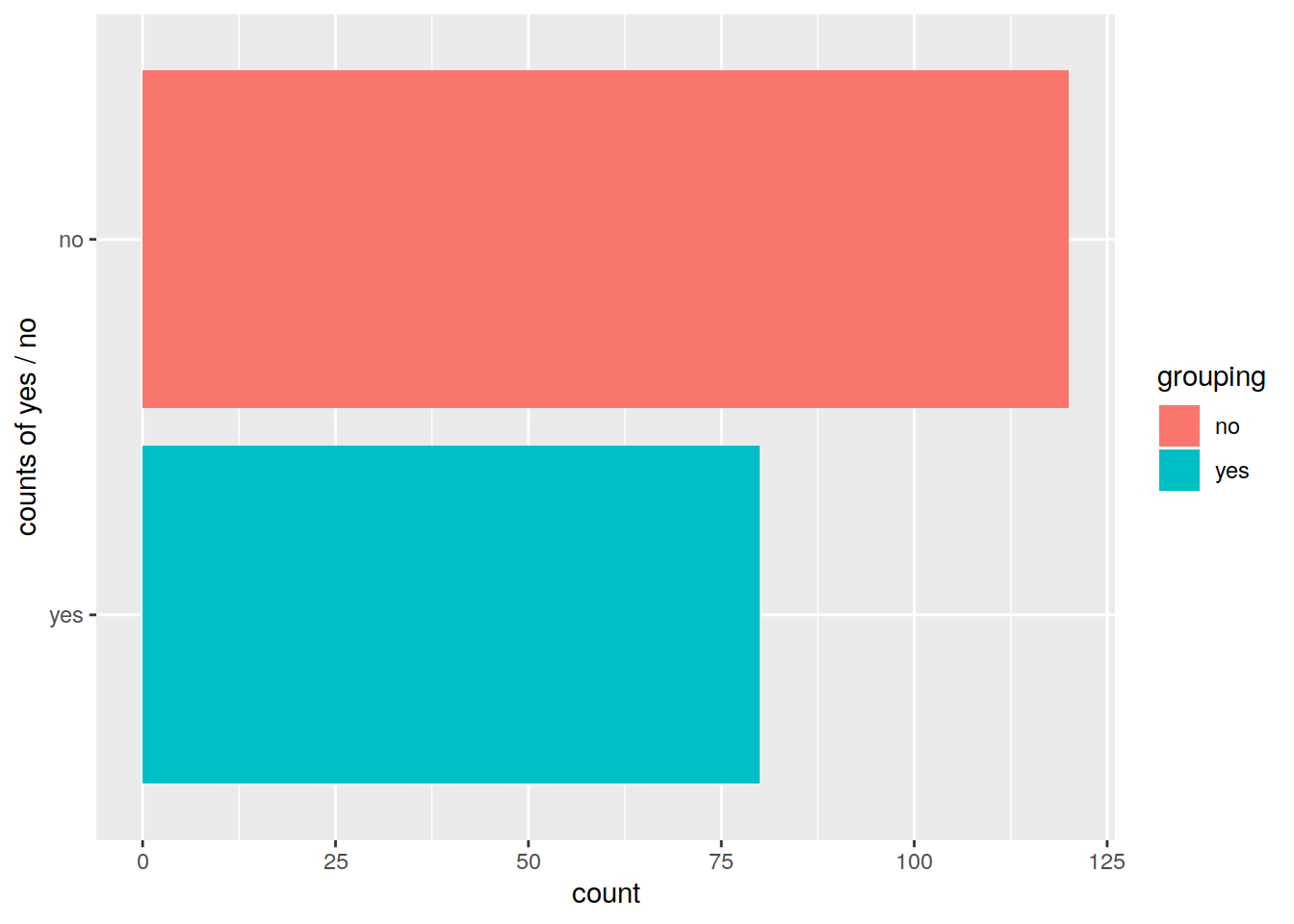``````# Change fill
p4 <- p3 + scale_fill_manual(values=c("yellow", "cyan"))

p4``````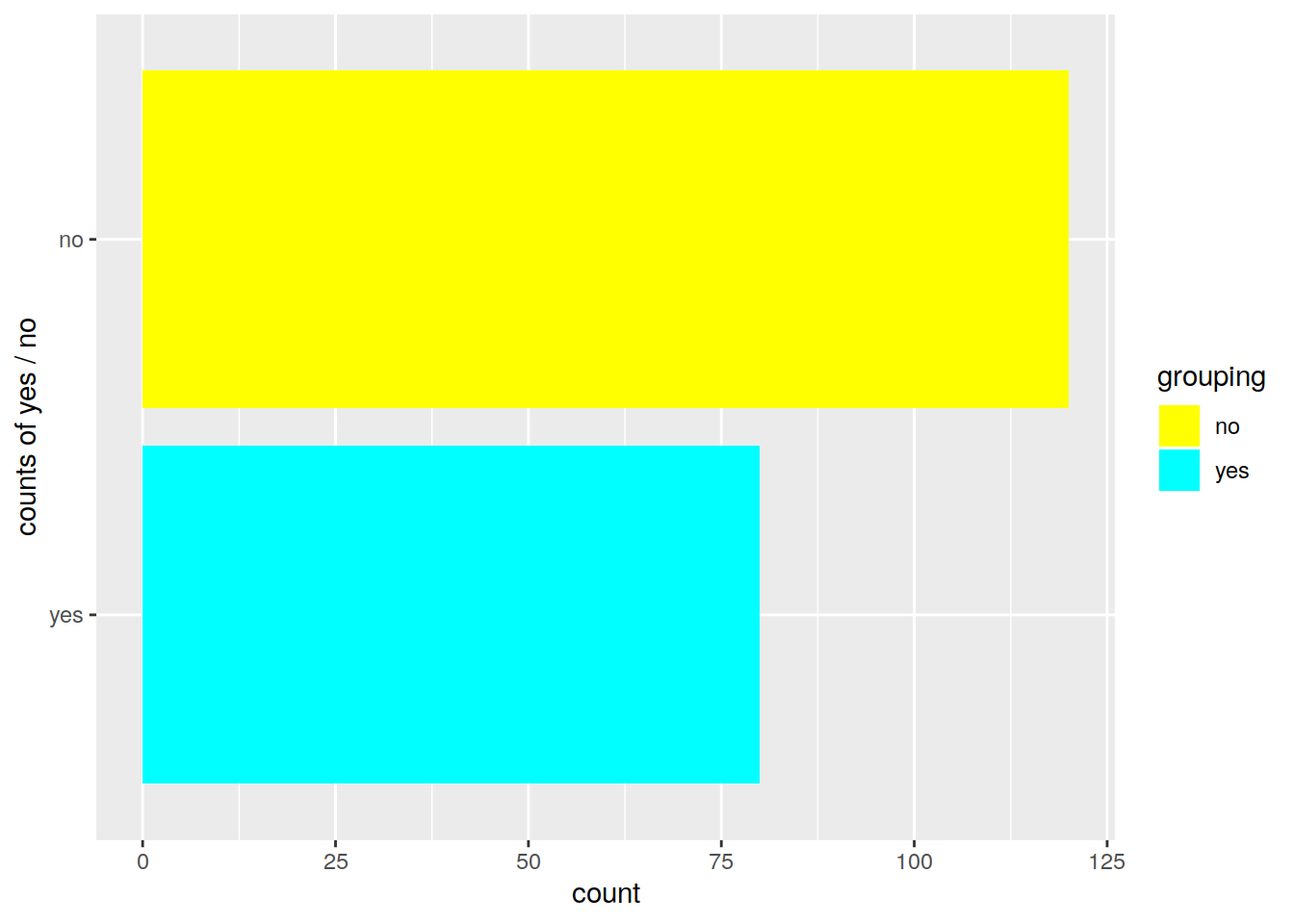### 19.5.1 Bar plots with error bars

We can create error bars on barplots.
Let’s create a toy data set, that contains 7 independent qPCR measurements for 3 genes:

``````pcr <- data.frame(Dkk1=c(18.2, 18.1, 17.8, 17.85, 18.6, 12.4, 10.7),
Pten=c(15.1,15.2, 15.0, 15.6, 15.3, 14.8, 15.9),
Tp53=c(9.1, 9.9, 9.25, 8.7, 8.8, 9.3, 7.8))``````

The height of the bar will represent the average qPCR measurement. The error bar will represent the average - standard deviation on the lowe part, and the average + standard deviation on the high part.
We need to create a data frame that summarizes these values:

``````pcr_summary <- data.frame(average=apply(pcr, 2, mean),
standard_deviation=apply(pcr, 2, sd),
genes=colnames(pcr))``````

And now we can plot!

``````ggplot(pcr_summary, aes(x=genes, y=average, fill=genes)) +
geom_bar(stat = "identity") +
geom_errorbar(aes(ymin=average-standard_deviation, ymax=average+standard_deviation), colour="black", width=.1) ``````